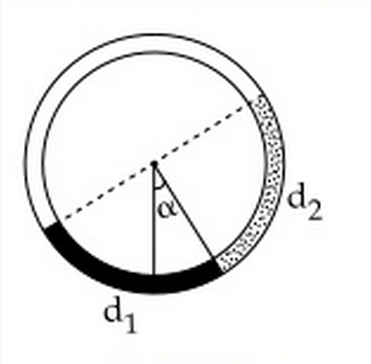# JEE-2015There is a circular tube in a vertical plane. Two liquids which do not mix and of densities $d_1$ and $d_2$ are filled in the tube. Each liquid subtends $90^\circ$ angle at centre. Radius joining their interface makes an angle $\alpha$ with vertical. Ratio $\frac {d_1}{d_2 }$ is:

×